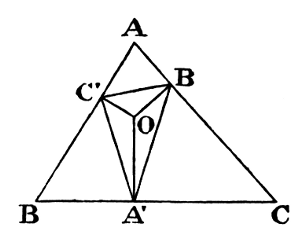#Pillow-Problems: Problem #4

Math Lair Home > Source Material > Pillow-Problems > Problem #4

## Problem:

4.

In a given acute-angled Triangle inscribe a Triangle, whose sides make, at each of the vertices, equal angles with the sides of the given Triangle.

[19/4/76

## Solution:

4.Let ABC be the given Triangle, and ABC′ the required Triangle, so that ∠ BAC′ = ∠CAB′, &c.

Evidently, AC′, AB′ are equally inclined to a line drawn, from A′, ⊥; BC; and so of the others: i.e. these ⊥;s bisect the ∠s at A′, B′, C′;

∴ they meet in the same Point. Draw them; let them meet at O; and call the ∠CAB′ ‘2α’, and so on.

Now (β + γ) = π − ∠BOC′ = A;

∴ 2A = 2(β + γ) = π − 2α

α = 90° − A;

∴ ∠BAC′ = A.

Similarly ∠BCA′ = C.

∴ Triangle BCA′ is similar to Triangle BCA; and so on of the others;

BA′ = ca · BC′ = ca · (cAC′),
= ca · (cbc · AB′),
= c²aba · (bCB′),
= c²ab²a+ ba · ab· CA′,
= c²ab²a + a - BA′;
∴ 2BA′ = c² + a² − b²a = 2ca cos Ba;
BA′ = c cos B;

A′ is foot of ⊥ drawn, from A, to BC. Hence the construction is obvious.

Q.E.F.